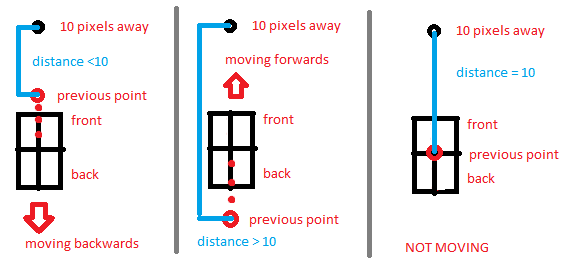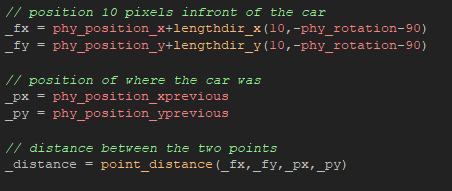# Math Issues - and why it's hard to sleep

So I have been re-writing my car logic today. I've spent a few hours trying to make the car behave like a real car.  I ran into an issue trying to figure out how to tell if the car was driving backwards (this was needed to steer the car differently when going backwards). I know it sounds simple but it isn't as straight forward as it seems. In Game Maker they give you variables for your physic objects like: speed, x and y velocity, rotation, etc. but no variable to tell you the relative direction you are travelling with relation to your rotation.

So I tried a few things. I created a vector using the x and y velocity and compared its angle to the rotation (angle) of the car. These measurements are given in degrees from 0 to 360. If the angles where the same then the car was traveling forward. The issue was the angles where never the same unless the car was not turning. This had to due with the drifting I programmed into the car. The car is not necessarily always pointing in the direction of movement. So I thought I wouldn't check for equality but for a range of values +-90 degrees. That worked! Except for when the degrees would switch from 360 to 0 (or vise versa) then the range would no longer be +-90 and then the condition would be wrong. Which would result in the car not turning or turn the opposite way. Ugh.

I tried lots of different approaches using all the functions and variables I could think of. The 360 to 0 issue kept popping up.

I finally came up with an answer. I decided to look at the problem from a different angle. Instead of comparing directions (which always seemed to give me an issue) I thought what else can I measure that is independent of angles? What is the other part of a vector... distance! So the solution was this:

1. Find a point exactly 10 (arbitrary distance) pixels away from the front of the car. This point does require a direction to calculate but the answer is only the x and y point along the vector ignoring angles (important because angles gave me issues)
2. Find a point using the physics variables xprevious and yprevious. These point are where the car was last frame. So if I was moving backwards the point would be in front of the car and if I was moving forward the point would be behind the car.
3. Then find out the distance from the first set of points and the second.
4. Because I am using the previous position I know if the distance between the points is less then 10; I am moving backwards. If it is more then 10 forwards. If it is 10 then I am stopped.CODE:This is a little insight of why I can't sleep at night :)